# Incenter

Incenter

In this mini-lesson, we will learn about the incenter of a triangle by understanding the properties of the incenter, the construction of the incenter, and how to apply them while solving problems. We will also discover interesting facts around them.

We all have seen triangles in our day to day life. We know that triangles have three sides and three angles, but what about other important components of the triangle.

Let's consider that Paul has a triangular field outside his house.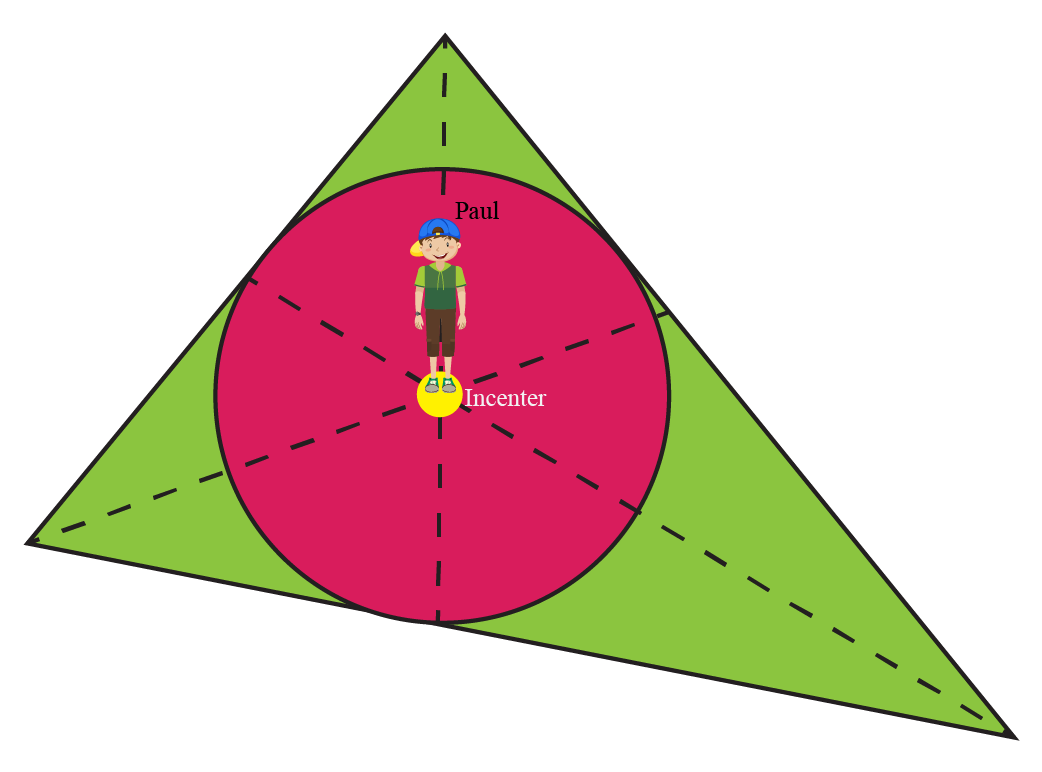Paul has divided all three angles equally and extended the lines.

All three lines intersect at one point.

So, Paul went to stand on that point.

Do you what is that point called?

Yes, Paul is standing on the incenter on the triangular field.

Let's explore the incenter of the triangle in more detail.

## Lesson Plan

 1 What Is the incenter of a triangle? 2 Thinking Out of the Box! 3 Solved Examples on Incenter 4 Important Notes on Incenter 5 Interactive Questions on Incenter

## What Is the Incenter of a Triangle?

The incenter of a triangle is the point of intersection of all the three interior angle bisectors of the triangle.

The angle bisectors in a triangle are always concurrent and the point of intersection is known as the incenter of the triangle.

Explore the simulation below to check out the incenters of different triangles.

The incenter of a triangle can also be explained as the center of the circle which is inscribed in a triangle $$\text{ABC}$$.

When a circle is inscribed in a triangle such that the circle touches each side of the triangle, the center of the circle is called the incenter of the triangle.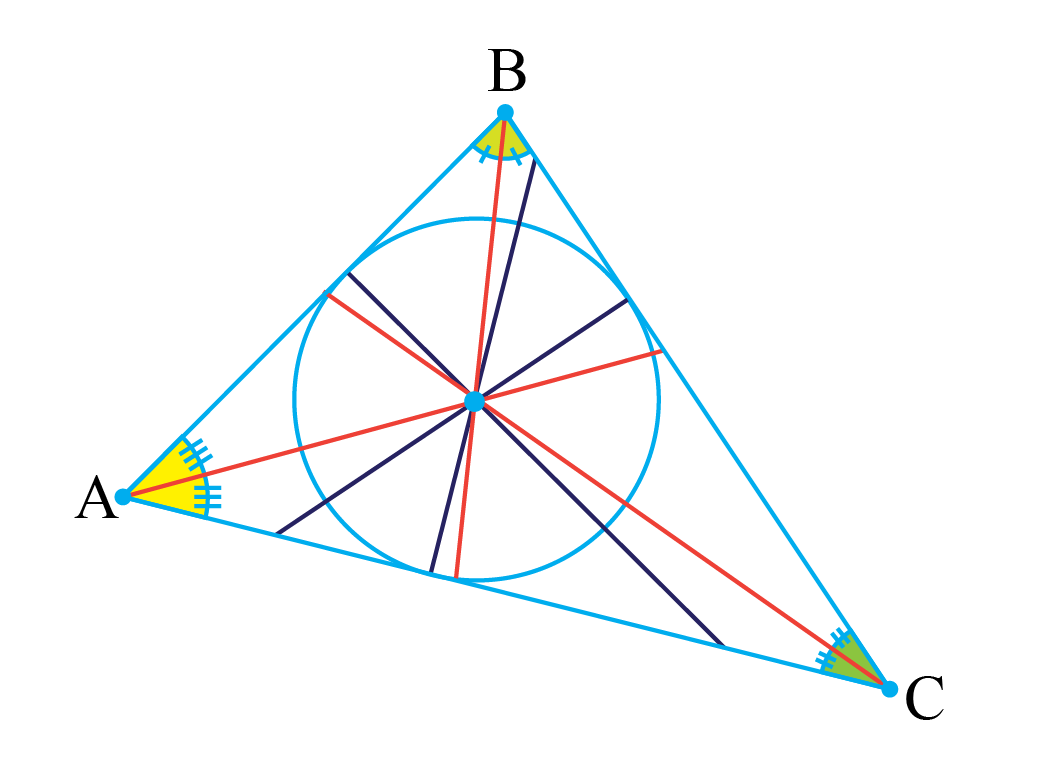## What Are Circumcenter, Centroid, and Orthocenter?

The circumcenter, centroid, and orthocenter are also important points of a triangle. Let's learn these one by one.

Circumcenter

The circumcenter of a triangle is the center of a circle which circumscribes the triangle.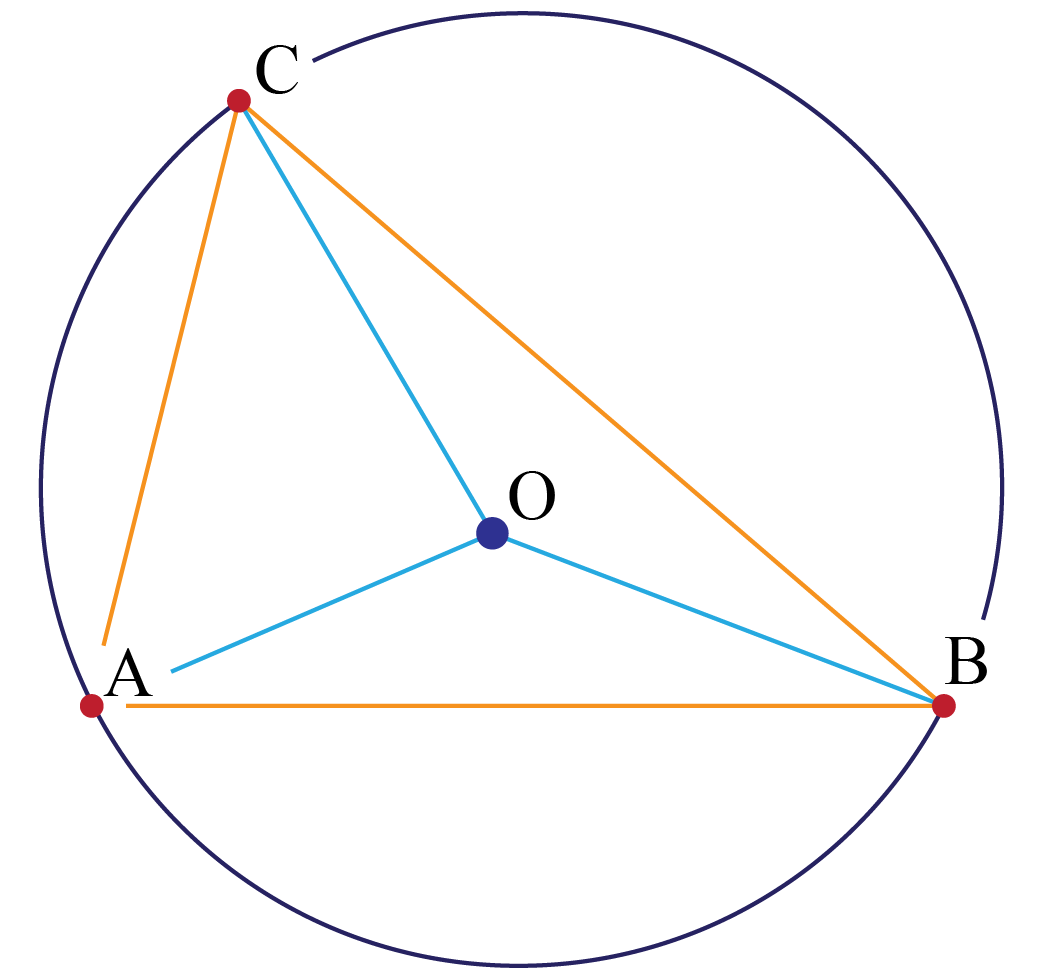Here $$\text{OA = OB = OC}$$, these are the radii of the circle.

The circumcenter of the triangle can also be described as the point of intersection of the perpendicular bisectors of each side of the triangle.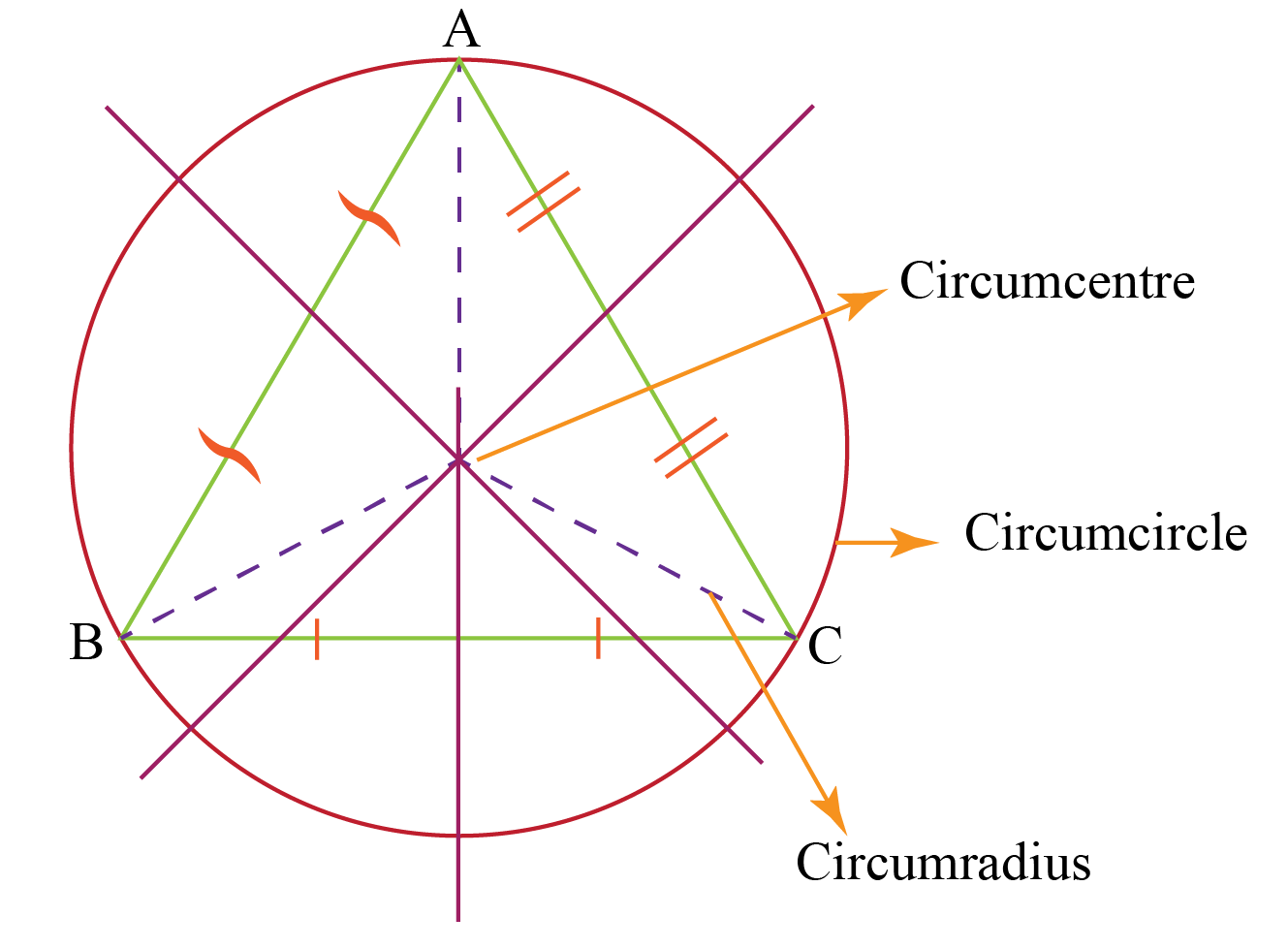Centroid

The centroid of a triangle is the point of intersection of all the three medians of the triangle.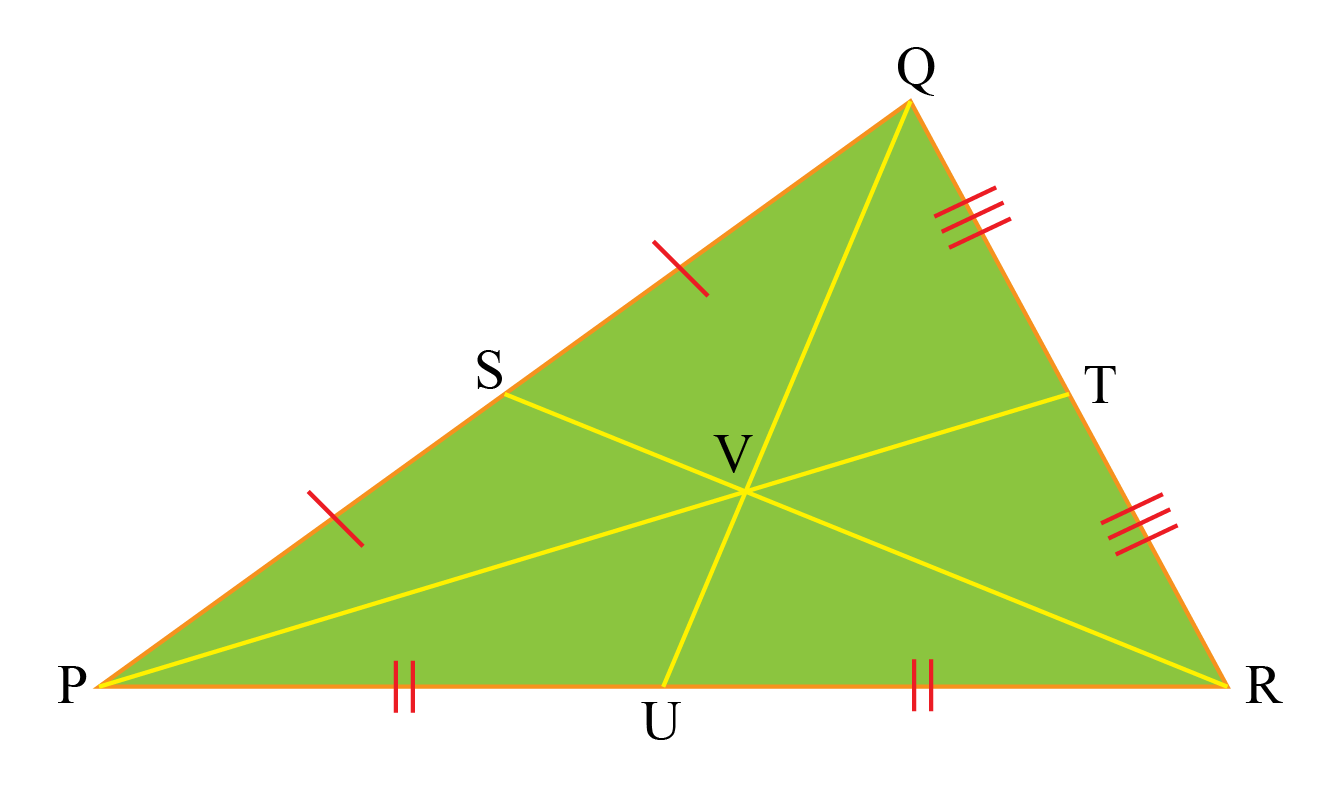In triangle $$\text{QRP}$$, point $$\text{V}$$ is the centroid of the triangle, and $$\text{QU}$$, $$\text{PT}$$, $$\text{RS}$$ are the median of the triangle.

$$\text{PU} = \text{UR} \$0.2cm] \text{RT} = \text{TQ} \\[0.2cm] \text{QS} = \text{SP}$$ Orthocenter The orthocenter of a triangle is the point of intersection of the perpendiculars dropped from each vertices to the opposite sides of the triangle.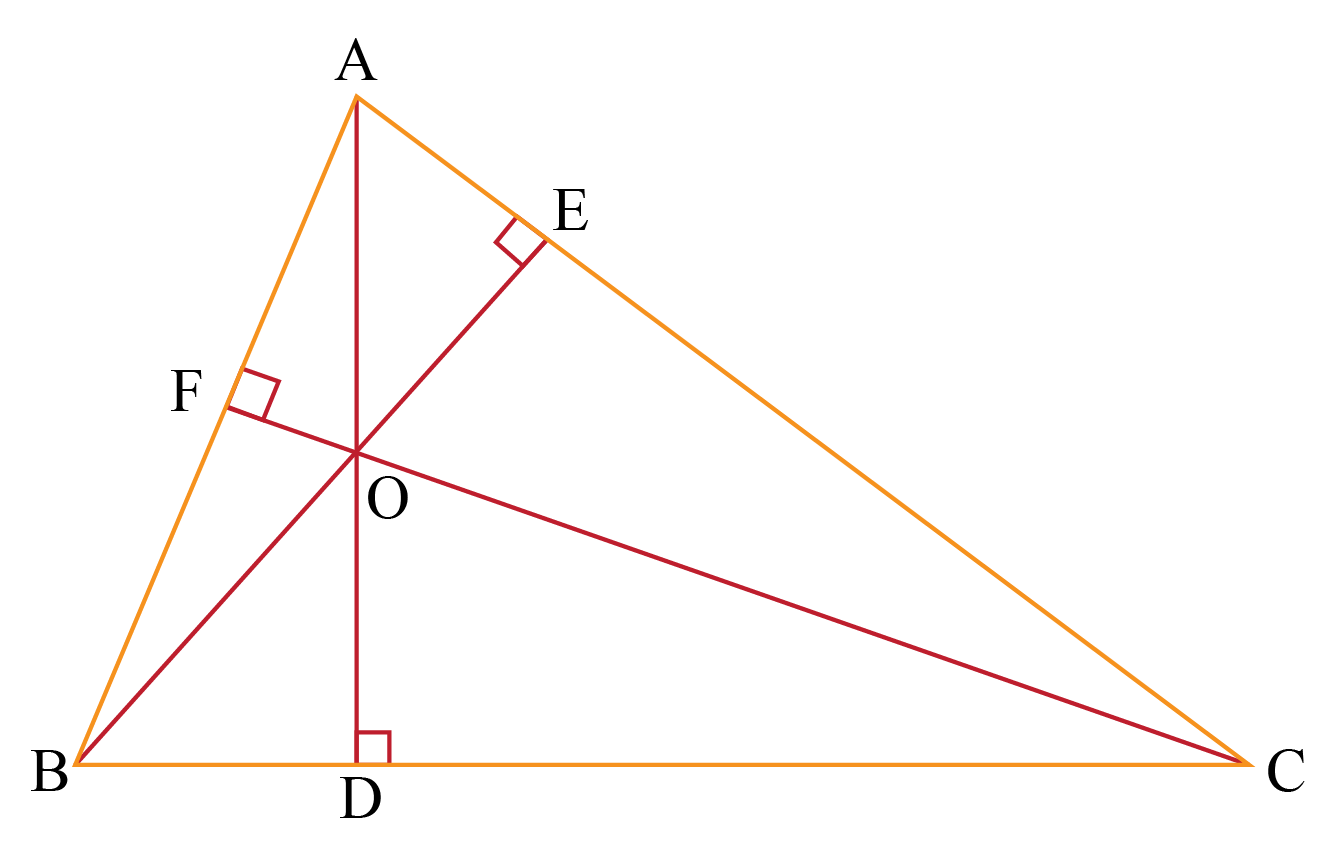$$\text{AD}, \text{BE}, \text{CF}$$ are the perpendiculars dropped from the vertex $$\text{A, B, and C}$$ to the sides $$\text{BC, CA, and AB}$$ respectively, of the triangle $$\text{ABC}$$. $$\text{O}$$ is the orthocenter of the triangle. ## What Are the Properties of an Incenter? Property 1: If $$\text{I}$$ is the incenter of the triangle then line segments AE and AG, CG and CF, BF and BE are equal in length.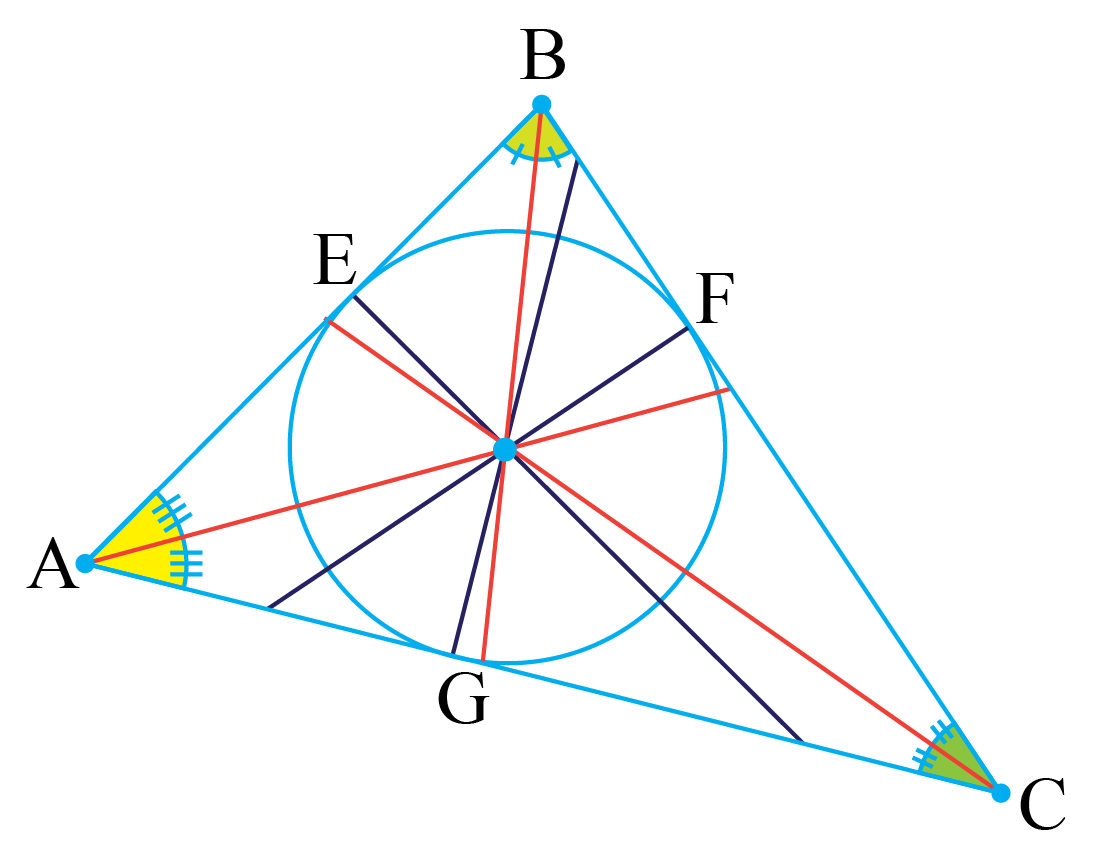Proof: The triangles $$\text{AEI}$$ and $$\text{AGI}$$ are congruent triangles by RHS rule of congruency. $$\text{AI} = \text{AI}$$ common in both triangles $$\text{IE} = \text{IG}$$ radius of the circle $$\angle \text{AEI} = \angle \text{AGI} = \text{90}^{\circ}$$ angles Hence $$\triangle \text{AEI} \cong \triangle \text{AGI}$$ So, by CPCT side $$\text{AE} = \text{AG}$$ Similarly, $$\text{CG} = \text{CF}$$ and $$\text{BF} = \text{BE}$$ Property 2: If $$\text{I}$$ is the incenter of the triangle, then $$\angle \text{BAI} = \angle \text{CAI}$$, $$\angle \text{ABI} = \angle \text{CBI}$$, and $$\angle \text{BCI} = \angle \text{ACI}$$. Proof: The triangles $$\text{AEI}$$ and $$\text{AGI}$$ are congruent triangles by RHS rule of congruency. We have already proved these two triangles congruent in the above proof. So, by CPCT $$\angle \text{BAI} = \angle \text{CAI}$$. Property 3: The sides of the triangle are tangents to the circle, hence $$\text{OE = OF = OG} = r$$ are called the inradii of the circle. Property 4: The coordinates of incenter of the triangle ABC with coordinates of the vertices, $$A(x_1, y_1), B(x_2, y_2), C(x_3, y_3)$$ and sides $$a, b, c$$ are:  \[(\dfrac{ax_1 + bx_2 + cx_3}{a + b + c}, \dfrac{ay_1 + by_2 + cy_3}{a + b + c})$

Property 5: If $$s = \dfrac{a + b + c}{2}$$, where $$s$$ is the semiperimeter of the triangle and $$r$$ is the inradius of the triangle, then the area of the triangle is:

 $\text{A} = sr$Think Tank
1. Is there any triangle possible for which all the four points: centroid, circumcenter, incenter, and orthocenter, coincide?
2. Is the above case possible for any isosceles or right-angle triangle?

## How to Construct the Incenter of a Triangle?

The construction of the incenter of a triangle is possible with the help of a compass.

The steps for construction can easily be understood with the help of the simulation below, explore it.

## Solved Examples

 Example 1

If $$I$$ is the incenter of the triangle $$\text{ABC}$$ then find the value of $$x$$ in the figure.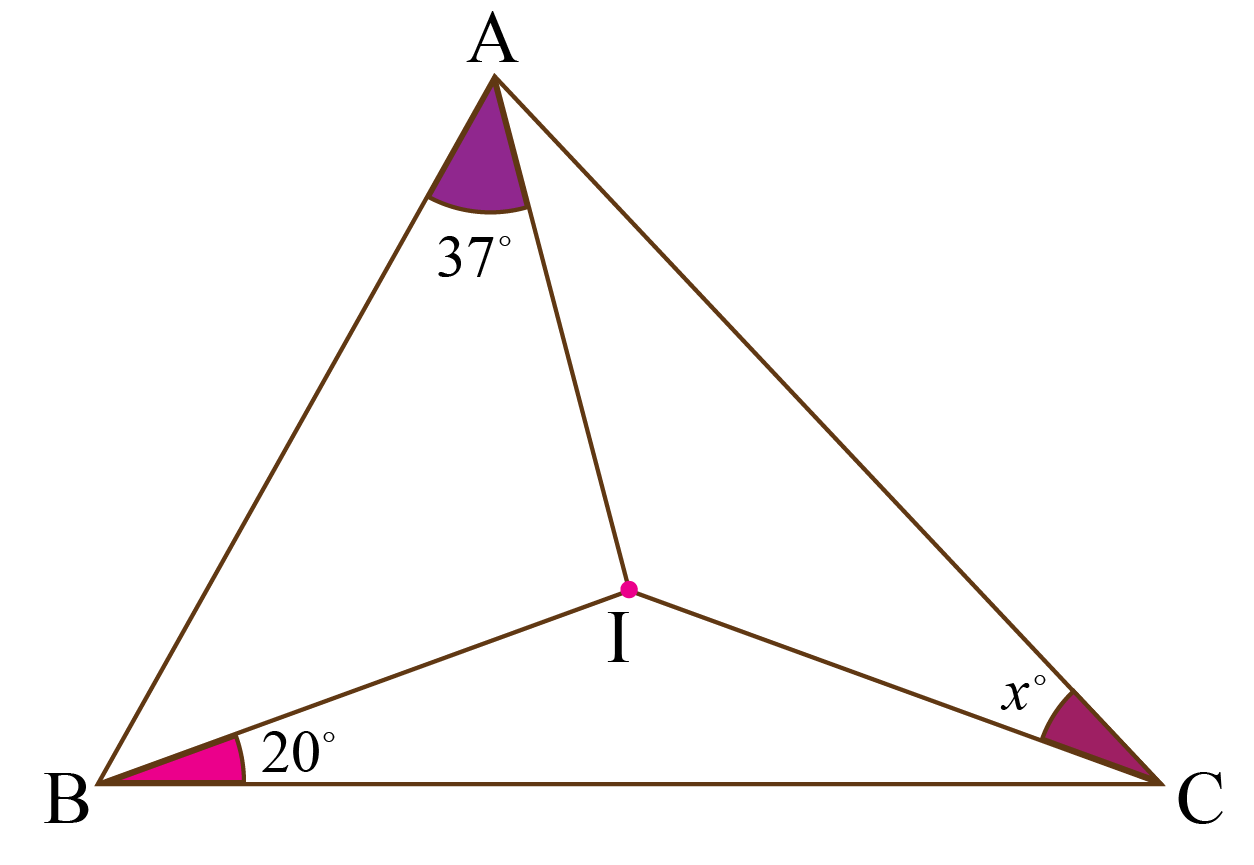Solution

Given:

$$I$$ is the incenter of the triangle.

$$\text{AI, BI, CI}$$ are the angle bisectors of the triangle, hence:

\begin{align}\angle \text{BAI} + \angle \text{CBI} + \angle \text{ACI} &= \frac{180^{\circ}}{2}\\[0.2cm] 37^{\circ} + 20^{\circ} + x^{\circ} &= 90^{\circ}\\[0.2cm] 57^{\circ} + x^{\circ} &= 90^{\circ}\\[0.2cm] x^{\circ} &= 90^{\circ} - 57^{\circ}\\[0.2cm] x^{\circ} &= 33^{\circ}\end{align}

 $\therefore x = 33^{\circ}$
 Example 2

Peter calculated the area of a triangular sheet as $$\text{90} \text{ feet}^{2}$$. The perimeter of the sheet is $$\text{30 feet}$$. If a circle is drawn inside the triangle such that it is touching every side of the triangle, help Peter calculate the inradius of the triangle.

Solution

Given:

The area of the sheet = $$\text{90} \text{ feet}^{2}$$

The perimeter of the sheet = $$\text{30 feet}$$

Semiperimeter of the triangular sheet = $$\dfrac{\text{30 feet}}{2} = \text{15 feet}$$

The area of the triangle = $$sr$$, where $$r$$ is the inradius of the triangle

\begin{align}\text{Area } &= sr \\[0.2cm] 90 &= 15 \times r \\[0.2cm] \dfrac{90}{15} &= r \\[0.2cm] 6 &= r \end{align}

 $\therefore r = 6 \text{ feet}$
 Example 3

The coordinates of the incenter of the triangle ABC formed by the points $$A(3, 1), B(0, 3), C(-3, 1)$$ is $$(p, q)$$. Find $$(p, q)$$.

Solution

Given:

The vertices of the triangles are $$A(3, 1), B(0, 3), C(-3, 1)$$

$$\text{c} = \text{AB} = \sqrt{{(3 - 0)}^{2} + {(1 - 3)}^{2}}$$

$$\text{c} = \text{AB} = \sqrt{{3}^{2} + {-2}^{2}} = \sqrt{\text{13}}$$

$$\text{a} = \text{BC} = \sqrt{{(-3 - 0)}^{2} + {(1 - 3)}^{2}}$$

$$\text{a} = \text{BC} = \sqrt{{-3}^{2} + {-2}^{2}} = \sqrt{\text{13}}$$

$$\text{b} = \text{AC} = \sqrt{{(-3 - 3)}^{2} + {(1 - 1)}^{2}}$$

$$\text{b} = \text{AC} = \sqrt{{-6}^{2} + {0}^{2}} = \text{6}$$

Incenter of the triangle is:

$(\dfrac{ax_1 + bx_2 + cx_3}{a + b + c}, \dfrac{ay_1 + by_2 + cy_3}{a + b + c})$
$(\dfrac{3 \sqrt{13} + 0 - 3 \sqrt{13}}{6 + 2 \sqrt{13}}, \dfrac{2 \sqrt{13} + 18}{6 + 2 \sqrt{13}})$
$(0, \dfrac{2 \sqrt{13} + 18}{6 + 2 \sqrt{13}})$

 $\therefore \text{Coordinates} = (0, \dfrac{2\sqrt{13} + 18}{6 + 2\sqrt{13}})$
More Important Topics
Numbers
Algebra
Geometry
Measurement
Money
Data
Trigonometry
Calculus